## Tamilnadu Samacheer Kalvi 12th Maths Solutions Chapter 6 Applications of Vector Algebra Ex 6.8

Question 1.
Show that the straight lines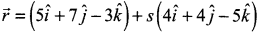and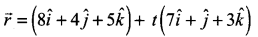are coplanar. Find the vector equation of the plane in which they lie.
Solution: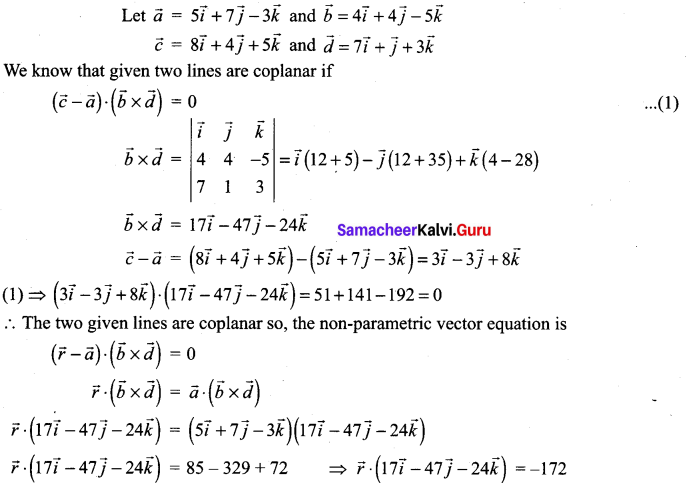Question 2.
Show that lines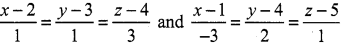are coplanar. Also, find the plane containing these lines.
Solution:
From the lines we have,
(x1, y1, z1) = (2, 3, 4) and (x2, y2, z2) = (1, 4, 5)
(b1, b2, b3) = (1, 1, 3) and (d1, d2, d3) = (-3, 2, 1)
Condition for coplanarity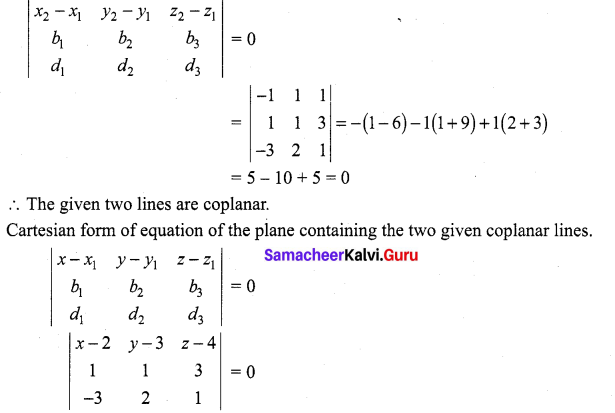(x – 2)[1 – 6] – (y – 3)[1 + 9] + (z – 4)[2 + 3] = 0
-5(x – 2) – 10(y – 3) + 5(z – 4) = 0
-5x + 10 – 10y + 30 + 5z – 20 = 0
-5x – 10y + 5z + 20 = 0
(÷ by -5) ⇒ x + 2y – 2z – 4 = 0Question 3.
If the straight lines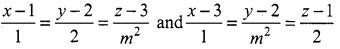are coplanar, find the district real values of m.
Solution:
From the lines we have,
(x1, y1, z1) = (1, 2, 3) and (x2, y2, z2) = (3, 2, 1)
(b1, b2, b3) = (1, 2, m2) and (d1, d2, d3) = (1, m2, 2)
Condition for coplanarity
$$\left|\begin{array}{ccc}{x_{2}-x_{1}} & {y_{2}-y_{1}} & {z_{2}-z_{1}} \\ {b_{1}} & {b_{2}} & {b_{3}} \\ {d_{1}} & {d_{2}} & {d_{3}}\end{array}\right|=0$$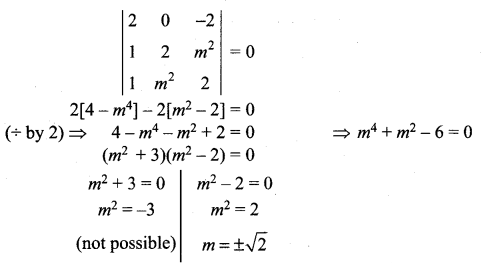Question 4.
If the straight lines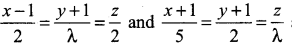are coplanar, find λ and equation of the planes containing these two lines.
Solution:
From the lines we have,
(x1, y1, z1) = (1, -1, 0) and (x2, y2, z2) = (-1, -1, 0)
(b1, b2, b3) = (2, λ, 2) and (d1, d2, d3) = (5, 2, λ)
Condition for coplanarity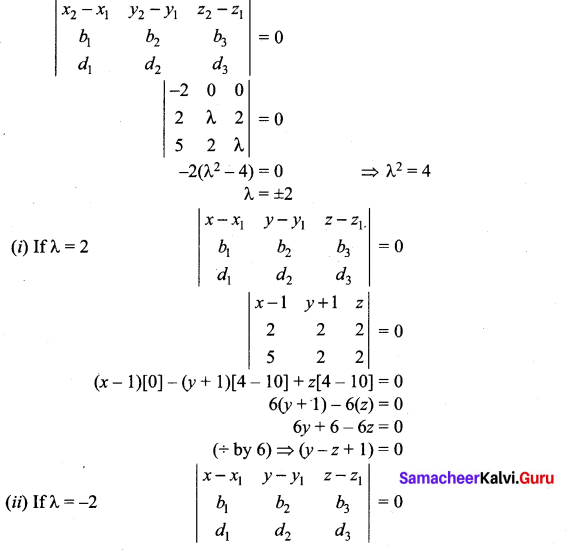$$\left|\begin{array}{ccc}{x-1} & {y+1} & {z} \\ {2} & {-2} & {2} \\ {5} & {2} & {-2}\end{array}\right|$$ = 0
(x – 1) – (y + 1)[-4 – 10] + z[4 + 10] = 0
14(y + 1) + 14z = 0 ⇒ 14y + 14 + 14z = 0
(÷ by 14) ⇒ y + z + 1 = 0

### Samacheer Kalvi 12th Maths Solutions Chapter 6 Applications of Vector Algebra Ex 6.8 Additional Problems

Question 1.
Show that the straight lines.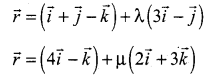are coplanar. Find the vector equation of the plane in which they lie.
Solution: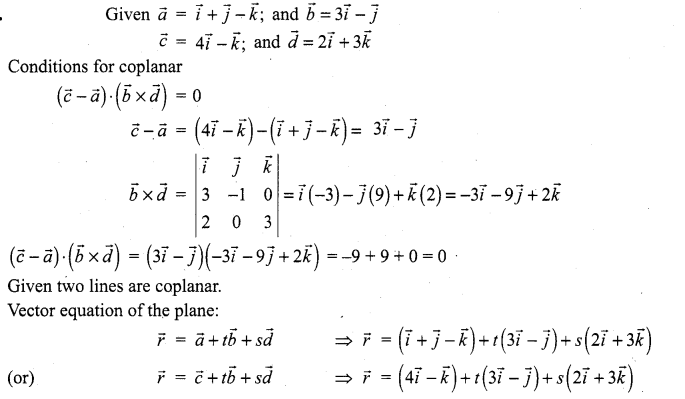Question 2.
If the straight lines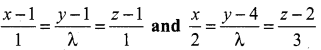are coplanar. Find λ.
Solution:
From the lines we have,
(x1, y1, z1) = (1, 1, 1) and (b1, b2, b3) = (1, λ, 1)
(x2, y2, z2) = (0, 4, 2) and (d1, d2, d3) = (2, λ, 3)
Condition for coplanarity
$$\left|\begin{array}{ccc}{x_{2}-x_{1}} & {y_{2}-y_{1}} & {z_{2}-z_{1}} \\ {b_{1}} & {b_{2}} & {b_{3}} \\ {d_{1}} & {d_{2}} & {d_{3}}\end{array}\right|$$ = 0
$$\left|\begin{array}{ccc}{-1} & {3} & {1} \\ {1} & {\lambda} & {1} \\ {2} & {\lambda} & {3}\end{array}\right|$$ = 0
-1(3λ – λ) – 3(3 – 2) + 1(λ – 2λ) = 0 ⇒ -2λ – 3 – λ = 0
-3λ = 3 ⇒ λ = -1

Question 3.
If the lines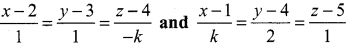are coplanar, then find the value of k.
Solution:
From the lines we have,
(x1, y1, z1) = (2, 3, 4) and (b1, b2, b3) = (1, 1, -1)
(x2, y2, z2) = (1, 4, 5) and (d1, d2, d3) = (k, 2, 1)
Condition for coplanarity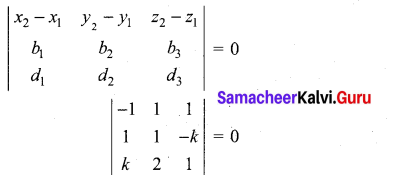-1(1 + 2k) – 1(1 + k2) + 1(2 – k) = 0
-1 – 2k – 1 – k2 + 2 – k = 0
-k2 – 3k = 0
k2 + 3k = 0
k(k + 3) = 0
k = 0 or k = -3Question 4.
Show that the lines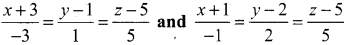are coplanar, Also find the equation of the plane containing these two lines.
Solution:
From the lines we have,
(x1, y1, z1) = (-3, 1, 5) and (b1, b2, b3) = (-3, 1, 5)
(x2, y2, z2) = (-1, 2, 5) and (d1, d2, d3) = (-1, 2, 5)
Condition for coplanarity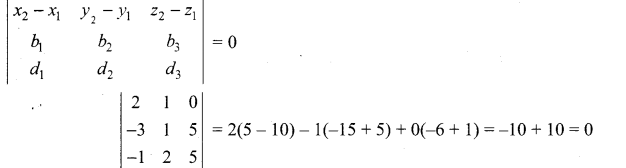Given two lines are coplanar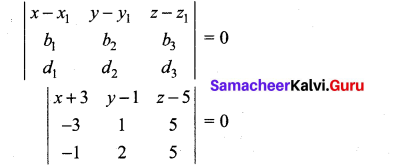(x + 3)[5 – 10] – (y – 1)[-15 + 5] + (z – 5)[-6 + 1] = 0
5(x + 3) + 10(y – 1) – 5(z – 5) = 0
(÷ by 5) ⇒ (x + 3) -2(y – 1) + (z – 5) = 0
x + 3 – 2y + 2 + z – 5 = 0
x – 2y + z = 0
or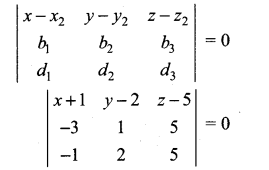(x + 1)(5 – 10) – (y – 2)(-15 + 5) + (z – 5)(-6 + 1) = 0
-5(x + 1) + 10(y – 2) – 5(z – 5) = 0
(x + 1) – 2(y – 2) + (z – 5) = 0
x + 1 – 2y + 4 + z – 5 = 0
x – 2y + z = 0